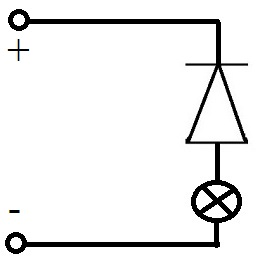# What are the different uses of diodes

## Diode: function and forward direction

We'll cover the basics of diodes in this article. It explains why a diode is actually needed and what the anode and cathode are all about. We look at the forward direction and reverse direction in a small circuit and thus the function / functionality of a diode. This article belongs to our field of physics or electrical engineering.

In this article we deal with diodes, or rather semiconductor diodes. This is a component that is very often used in electrical engineering and that can be found in many circuits. The special thing about a diode is that it lets the current through very easily in one direction and blocks it in the other direction (this is called insulation). There is also no such thing as "one diode", but rather different types of diodes, with which you can realize different effects particularly well.

Show:

### Possible uses for diodes

Here is a small selection of things that can be done with different types of diodes:

• A diode can be used to block the current in a certain direction.
• Tensions can be limited with this so that a component cannot be destroyed by overvoltage.
• Diodes are used to convert alternating voltage into direct voltage. One speaks here of rectification.
• Voltages can be stabilized (with Zener diodes).

In electrical engineering, circuit diagrams are used to show which components are used and how they are connected to one another. The Circuit symbols a "simple" diode consists of a triangle and a line and looks like this: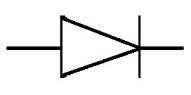Sometimes you write on the diodes where the anode and cathode are. This is usually abbreviated as A for anode and K for cathode. The cathode is on the line.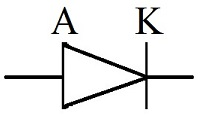If you hold a diode in your hand, it usually doesn't have A or K on it. Instead you can see a line on this one. The line on the housing indicates that the cathode is on this side.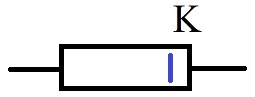Show:

### Diode: flow direction and reverse direction

In the introduction it was already described that one can block the current in one direction with a diode and let it through in the other direction. This is exactly what is to be shown here by means of an example. We have a small circuit with a positive pole and a negative pole, a light bulb and a diode. Depending on how the diode is switched, the light bulb lights up or it doesn't light up.

Diode in forward direction (light bulb lights up):

Let's start with the diode in the forward direction. Here the anode is connected to the positive pole of the voltage source, the light bulb lights up.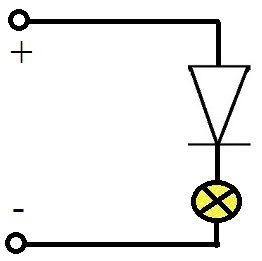Reverse diode (Light bulb does not light):

Now let's flip the diode. This means that the cathode is connected to the positive pole. The diode blocks, the lamp does not light up.